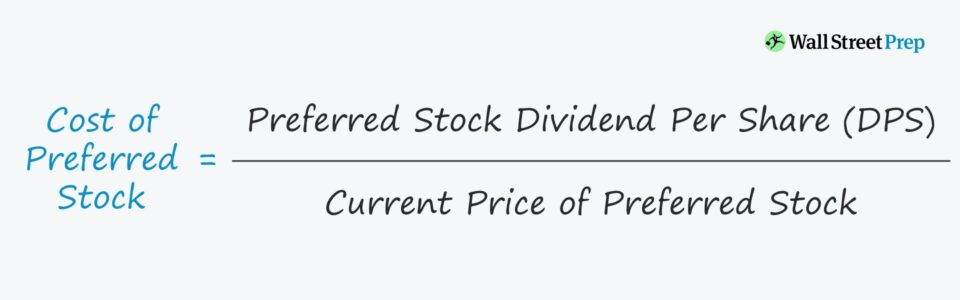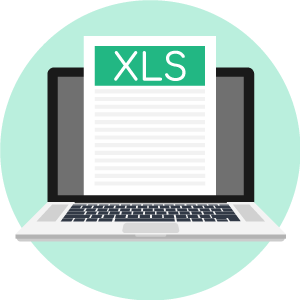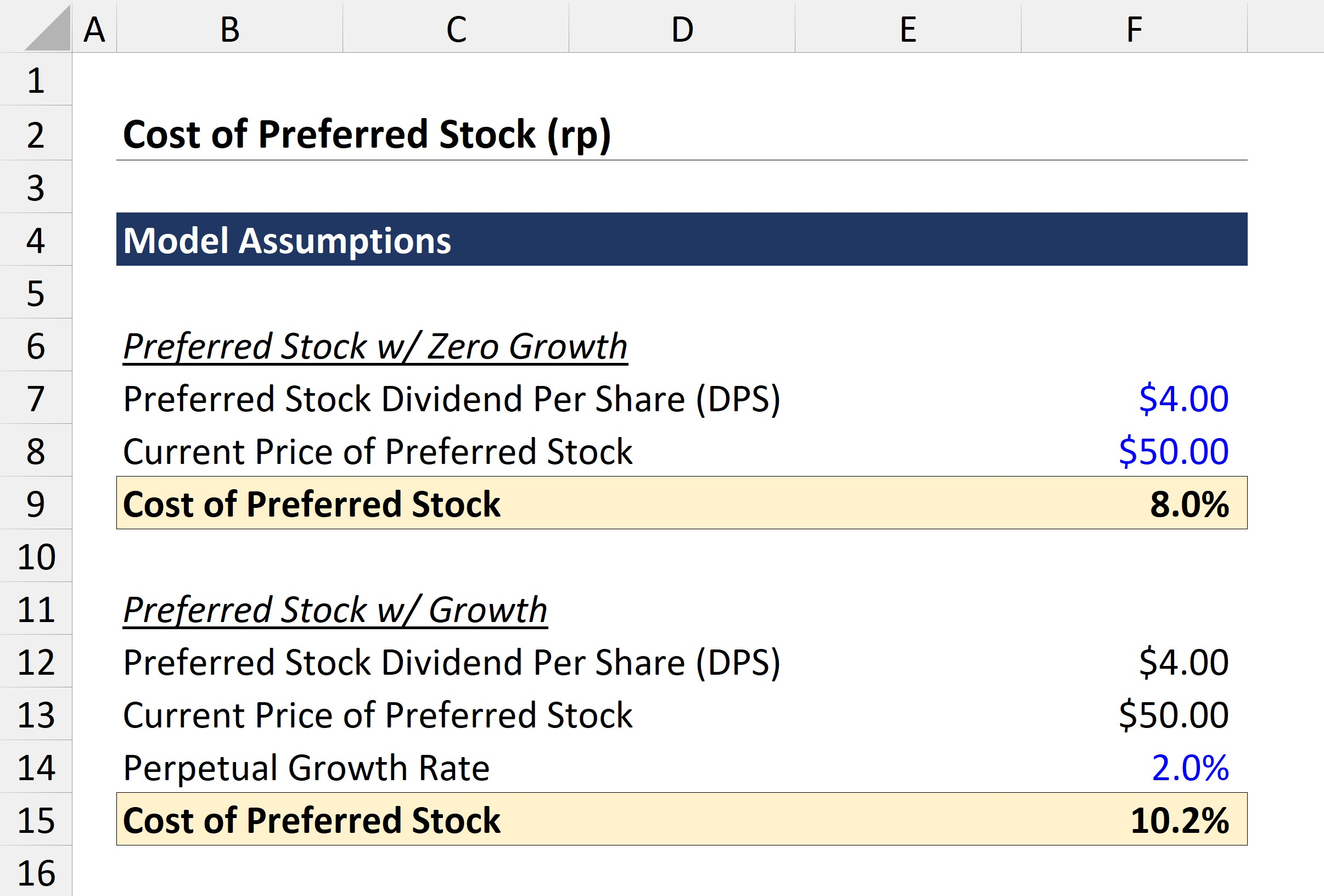# Cost of Preferred Stock (rp)

Guide to Understanding the Cost of Preferred Stock• What does the cost of preferred stock represent?
• Which formula is used to calculate the cost of preferred stock?
• Is the cost of preferred stock higher or lower than the cost of equity (and cost of debt)?
• How does growth in the dividend per share (DPS) impact the cost of preferred stock?

## Cost of Preferred Stock Definition

The recommended modeling best practice for hybrid securities such as preferred stock is to treat it as a separate component of the capital structure.

But one common point of confusion is, “Why should preferred stock be separated from equity and debt in the first place?”

Preferred equity is not quite debt capital nor is it common equity, so it has unique attributes that warrant it being a separate input in the WACC formula.

The cost of preferred equity, barring unusual circumstances, typically does not have a material impact on the ultimate firm valuation.

Thus, if the preferred equity amount is minuscule, it could be lumped together with debt, and the net impact on the valuation is going to be marginal.

Nevertheless, preferred stock should still be properly accounted for in a proper calculation of firm value.

## Cost of Preferred Stock vs Cost of Equity / Debt

In the capital structure, preferred stock sits in between debt and common equity – and these are the three key inputs for the cost of capital (WACC) calculation.

All debt instruments – regardless of the risk profile (e.g. mezzanine debt) – are of higher seniority than preferred stock.

On the other hand, preferred stock is senior to common stock and a company cannot legally issue a dividend to common shareholders without also issuing dividends to preferred shareholders.

Most preferred stock is issued without a maturity date, as mentioned earlier (i.e. with perpetual dividend income). However, note that there are instances when companies issue preferred stock with a fixed maturity date.

Additionally, unlike the interest expense associated with debt capital, the dividends paid on preferred stock are NOT tax-deductible, as with common dividends.

## Cost of Preferred Stock Formula

The cost of preferred stock represents the dividend yield on the preferred equity securities issued.

The cost of preferred stock is equal to the preferred stock dividend per share (DPS) divided by the issuance price per preferred share.

###### Cost of Preferred Stock Formula
• Cost of Preferred Stock = Preferred Stock Dividend Per Share (DPS) / Current Price of Preferred Stock

Similar to common stock, preferred stock is typically assumed to last into perpetuity – i.e. with an unlimited useful life and a forever-ongoing fixed dividend payment.

Hence, the cost of preferred stock is analogous to the perpetuity formula as used in the valuation of bonds and debt-like instruments.

As for the dividend per share (DPS), the amount is ordinarily specified as a percentage of the par value or as a fixed amount.

The formula for calculating the cost of preferred stock is the annual preferred dividend payment divided by the current share price of the stock.

In this case, we are assuming the most straightforward variation of preferred stock, which comes with no convertibility or callable features.

The value of preferred stock is equal to the present value (PV) of its periodic dividends (i.e. the cash flows to preferred shareholders), with a discount rate applied to factor in the risk of the preferred stock and the opportunity cost of capital.

Upon re-arranging the formula, we can arrive at the formula in which the cost of preferred stock (i.e. the discount rate) is equal to the preferred DPS divided by the current price of the preferred stock.

If dividend growth is expected, then the following formula would be used instead:In the numerator, we project the growth in the preferred stock DPS for one year using the growth rate assumption, divide by the price of the preferred stock, and then add the perpetual rate (g), which refers to the anticipated growth in the preferred DPS.

###### Example Calculation of Cost of Preferred Stock

Let’s say a company has issued “vanilla” preferred stock, on which the company issues out a fixed dividend of \$4.00 per share.

If the current price of the company’s preferred stock is \$80.00, then the cost of preferred stock is equal to 5.0%.

• Cost of Preferred Stock = \$4.00 / \$80.00 = 5.0%

## Nuances to the Cost of Preferred Stock

Sometimes, preferred stock is issued with additional features that ultimately impact its yield and the cost of the financing.

For instance, preferred stock can come with call options, conversion features (i.e. can be converted into common stock), cumulative paid-in-kind (PIK) dividends, and more.

Discretion is required in such cases, as there is no precise methodology for treating these features due to the amount of uncertainty that cannot all be accounted for when estimating the cost of the preferred stock.

Based on the most probable outcome, which is highly subjective, you’ll need to make adjustments as seen fit – e.g. when dealing with preferred equity with convertible features, the security could be broken into separate debt (straight-debt treatment) and equity (conversion option) components.

## Cost of Preferred Stock Calculator – Excel Template

We’ll now move to a modeling exercise, which you can access by filling out the form below.Submitting ...

## Cost of Preferred Stock Example Calculation

In our modeling exercise, we’ll be calculating the cost of preferred stock for two different dividend growth profiles:

1. Zero Growth in Dividend Per Share (DPS)
2. Perpetual Growth in Dividend Per Share (DPS)

For each scenario, the following assumptions will remain constant:

###### Model Assumptions
• Preferred Stock Dividend Per Share (DPS) = \$4.00
• Current Price of Preferred Stock = \$50.00

In the first type of preferred stock, there is no growth in the DPS.

Therefore, we enter our numbers into the simple cost of preferred stock formula to get the following:

• Cost of Preferred Stock = \$4.00 / \$50.00 = 8.0%

As for the next type of preferred stock, the assumption here is that DPS will grow at a perpetual rate of 2.0%.

The formula used to calculate the cost of preferred stock with growth is as follows:

• Cost of Preferred Stock = [\$4.00 * (1 + 2.0%) / \$50.00] + 2.0%

The formula above tells us that the cost of preferred stock is equal to the expected preferred dividend amount in Year 1 divided by the current price of the preferred stock, plus the perpetual growth rate.

Since the preferred stock is expected to grow at a fixed growth rate, which is 2.0% in our example, the cost of preferred stock is higher than in the case with zero DPS – as investors would expect a higher rate of return, which would directly impact the pricing of the shares.Step-by-Step Online Course

#### Everything You Need To Master Financial Modeling

Enroll in The Premium Package: Learn Financial Statement Modeling, DCF, M&A, LBO and Comps. The same training program used at top investment banks.Inline FeedbacksNurbol
June 9, 2022 9:07 am

do the preferred stocks dilute the shares amount during valuation?Learn Financial Modeling Online

Everything you need to master financial and valuation modeling: 3-Statement Modeling, DCF, Comps, M&A and LBO.

X

The Wall Street Prep Quicklesson Series

7 Free Financial Modeling Lessons

Get instant access to video lessons taught by experienced investment bankers. Learn financial statement modeling, DCF, M&A, LBO, Comps and Excel shortcuts.